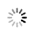# 如何在pandas dataframe 中组合AND和OR运算符？

3月前 ⋅ 37 阅读

### ChatGPT的回答仅作参考：

```在pandas dataframe 中组合AND和OR运算符，可以使用圆括号来明确运算顺序。例如：

```python
import pandas as pd

df = pd.DataFrame({'A': [1, 2, 3, 4, 5], 'B': [6, 7, 8, 9, 10], 'C': [11, 12, 13, 14, 15]})

# 组合AND和OR运算符
df[(df['A'] > 2) & ((df['B'] < 8) | (df['C'] > 13))]
```

### 相关推荐